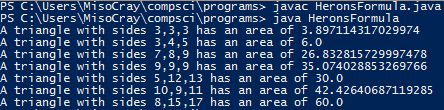# Assignemnt #92 Herons Formula

## Code

```    /// Name: Drew Boxold
/// Period 5
/// Program Name: Herons Formula
/// File Name: HeronsFormula.java
/// Date Finished: 4/1/16

public class HeronsFormula
{
public static void main( String[] args )
{
double a;

a = triangleArea(3, 3, 3);
System.out.println("A triangle with sides 3,3,3 has an area of " + a );

a = triangleArea(3, 4, 5);
System.out.println("A triangle with sides 3,4,5 has an area of " + a );

a = triangleArea(7, 8, 9);
System.out.println("A triangle with sides 7,8,9 has an area of " + a );

a = triangleArea(9, 9, 9);
System.out.println("A triangle with sides 9,9,9 has an area of " + a );
// It was easy to add another method call because I only had to copy paste and change the numbers

System.out.println("A triangle with sides 5,12,13 has an area of " + triangleArea(5, 12, 13) );
System.out.println("A triangle with sides 10,9,11 has an area of " + triangleArea(10, 9, 11) );
System.out.println("A triangle with sides 8,15,17 has an area of " + triangleArea(8, 15, 17) );
}

public static double triangleArea( int a, int b, int c )
{
// the code in this method computes the area of a triangle whose sides have lengths a, b, and c
double s, A;

s = (a+b+c) / 2.0;
A = Math.sqrt( s*(s-a)*(s-b)*(s-c) );

return A;
// ^ after computing the area, "return" it

/*
1. Both files produce the same output.
2. HeronsFormula.java is 32 lines. HeronsFormulaNoMethod is 50 lines.
3. The java file with the method was much easier to fix than with no method.
*/
}
}
```

### Picture of the output